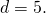Midterm 1 Preparation

# Midterm 1: Version C

1. Evaluate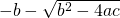if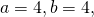and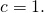2. Solve for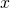in the equation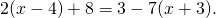3. Isolate the variable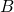in the equation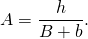4. Solve forin the equation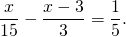5. Write the equation of the vertical line that passes through the point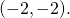6. Find the equation that has a slope of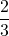and passes through the point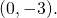7. Find the equation of the line passing through the points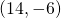and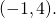8. Graph the relation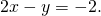For questions 9 to 11, find each solution set and graph it.

1.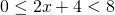2.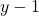>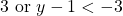3.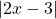>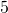4. Graph the relation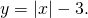5. Find two numbers such that 5 times the larger number plus 3 times the smaller is 49, and 4 times the larger minus twice the smaller is 26.
6. Karl is going to cut a 42 cm cable into 2 pieces. If the first piece is to be 5 times as long as the second piece, find the length of each piece.
7.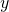varies jointly with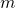and inversely with the square of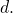If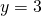when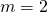and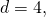find the constant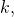then use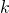to findwhen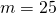and